# ACT Math - Coordinate Geometry: Tutoring Solution Chapter Exam

Exam Instructions:

Choose your answers to the questions and click 'Next' to see the next set of questions. You can skip questions if you would like and come back to them later with the yellow "Go To First Skipped Question" button. When you have completed the practice exam, a green submit button will appear. Click it to see your results. Good luck!

### Page 1

#### Question 1 1. Find the center and radius of the following equation: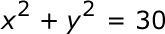### Page 2

#### Question 7 7. If the graph below was written in the slope-intercept form, which of the following would be true?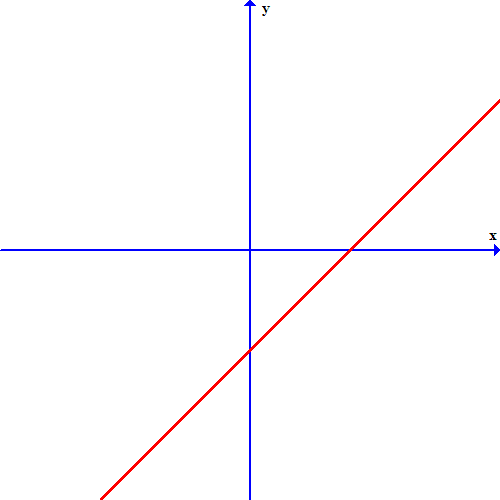#### Question 9 9. Find the center and radius of the following equation: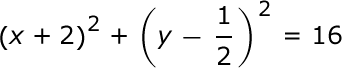#### Question 10 10. Which equation matches the graph below?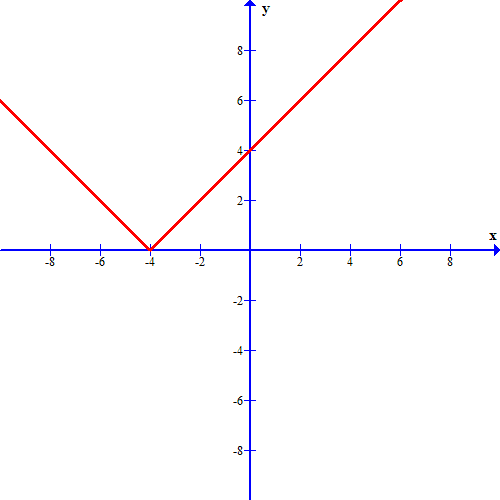### Page 3

#### Question 12 12. Choose the equation for the following circle: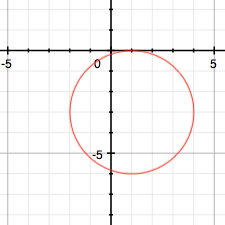### Page 4

#### Question 17 17. If the graph below has the equation Ax+By=C, which statement MUST be true?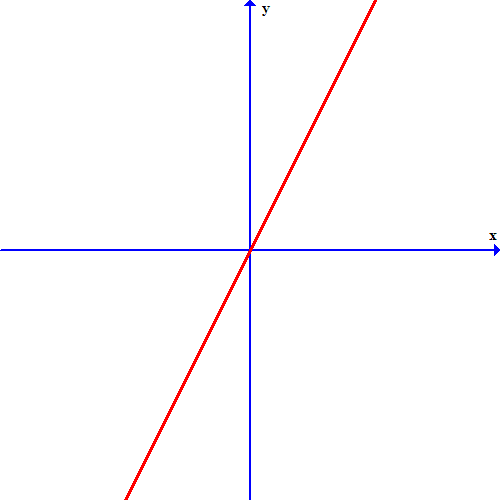#### Question 18 18. Which one of the following equations could work for the following graph?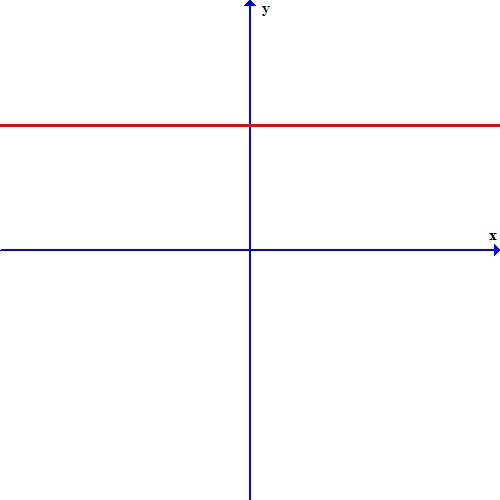### Page 5

#### Question 21 21. Which equation describes the following graph?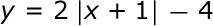#### Question 22 22. Find the center and radius of the following equation: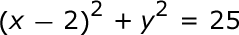#### Question 24 24. What is the slope of the line tangent to the curve given by the equation y = (x-1)²+2 at the point (1,2)?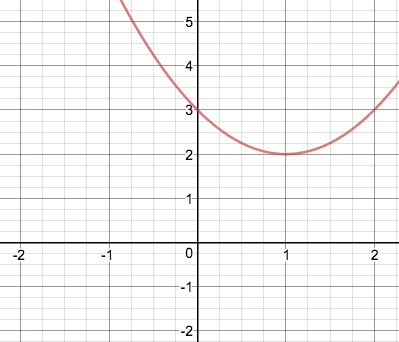### Page 6

#### Question 27 27. What are the coordinates of this point?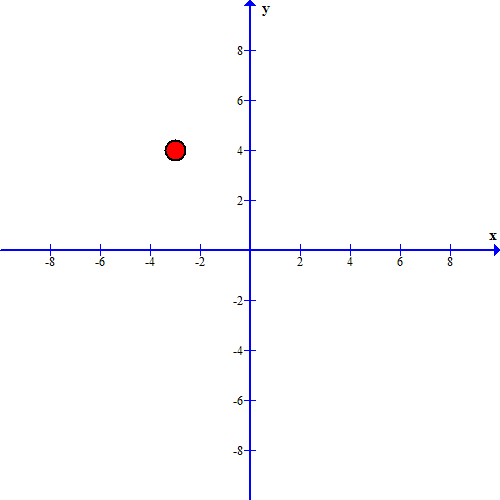#### Question 28 28. Estimate the coordinates of the midpoint of the line segment below: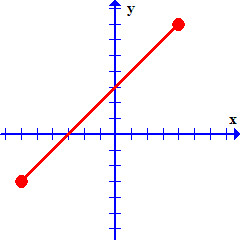#### ACT Math - Coordinate Geometry: Tutoring Solution Chapter Exam Instructions

Choose your answers to the questions and click 'Next' to see the next set of questions. You can skip questions if you would like and come back to them later with the yellow "Go To First Skipped Question" button. When you have completed the practice exam, a green submit button will appear. Click it to see your results. Good luck!

Support